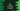# C program to check if a string is empty or not## C program to check if a string is empty or not:

In this post, we will learn how to check if a string is empty in C programming language. We will learn two different ways to check if a string is empty. C doesn’t provide any inbuilt method to check for empty strings. We can either check the total count of characters or we can check if the first character is a null character.

Let’s try each methods with an example for each.

### Method 1: Check for string length to find if the string is empty:

In this method, we will check the length of the string. If it is 0, the string is empty. Else, it is not. To get the length of a string, we can use the strlen method. This method takes one string as its argument and returns the length for that string. If the return value of this function is 0, then the string is empty. Else, it is not.

``````#include <stdio.h>
#include <string.h>

int isStringEmpty(char *str)
{
if (strlen(str) == 0)
{
return 0;
}
return 1;
}
int main()
{
char given_str[] = "";
char given_str2[] = "Hello";

if (isStringEmpty(given_str) == 0)
{
printf("given_str is empty\n");
}
else
{
printf("given_str is not empty\n");
}

if (isStringEmpty(given_str2) == 0)
{
printf("given_str2 is empty\n");
}
else
{
printf("given_str2 is not empty\n");
}
}``````

In this program,

• given_str is an empty string and given_str2 is a non-empty string.
• isStringEmpty method is used to check if a string is empty or not. This method returns one integer. It returns 0 if it is empty, else it returns 1.
• isStringEmpty checks the size of the string by using strlen method. It checks the output of this method. If it is 0, i.e. string is empty, it returns 0. Else, it returns 1.
• We are calling this method with given_str and given_str2 and printing one message for each.

If you run this program, it will print the below output:

``````given_str is empty
given_str2 is not empty``````

### Method 2: Check if the first character is a null character:

We can also check if the first character of a string is a null character or not, i.e. if it is \0 or not. All strings in C are terminated with a null character. So, if the first character is null, it means that this is a empty string.

Let’s try to modify the above program.

``````#include <stdio.h>
#include <string.h>

int isStringEmpty(char *str)
{
if (str == '\0')
{
return 0;
}
return 1;
}
int main()
{
char given_str[] = "";
char given_str2[] = "Hello";

if (isStringEmpty(given_str) == 0)
{
printf("given_str is empty\n");
}
else
{
printf("given_str is not empty\n");
}

if (isStringEmpty(given_str2) == 0)
{
printf("given_str2 is empty\n");
}
else
{
printf("given_str2 is not empty\n");
}
}``````

Here,

• I made changes to the isStringEmpty method.
• It checks the first chracter of the string. The index of the first character is 0, so str gives the first character.
• It is checking if the first character is equal to \0 or not. If it is, it returns 0. Else, it returns 1.

If you run this program, it will print the same output.